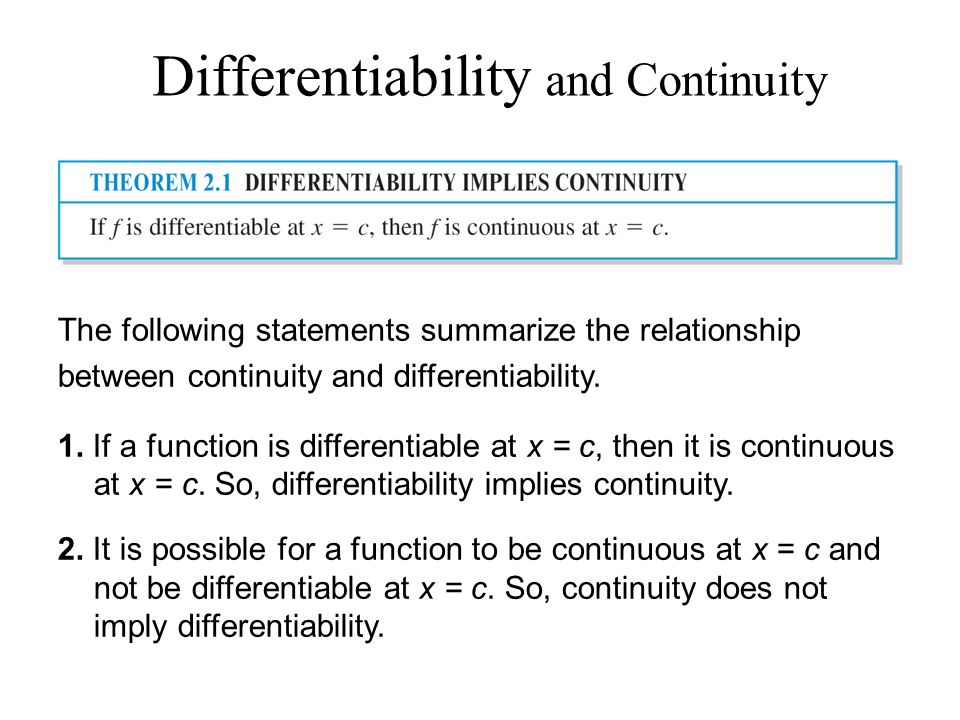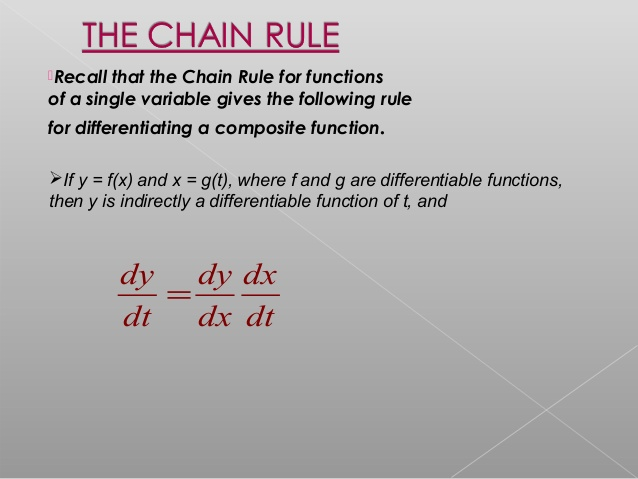Mathematics Differentiablity, Derivative of composite function and Chain Rule For CBSE-NCERT
Click for Only Video

### Topic covered

star Differentiability
star Theorem : If A function f is differentiable at a point c, then it is also continuous at that point.
star Derivatives of composite functions
star Theorem : (Chain Rule)

### Differentiability● If f is a real function and c is a point in its domain. The derivative of f at c is defined by color{red}{lim_(h-> 0) (f(c+h)-f(c))/h}

●So a function f is differentiable at a point c in its domain if both lim_(h-> 0^-) (f(c+h)-f(c))/h and lim_(h-> 0^+) (f(c+h)-f(c))/h are finite and equal.

color{blue}{lim_(h-> 0^-) (f(c+h)-f(c))/h = lim_(h-> 0^+) (f(c+h)-f(c))/h}

●Derivative of f at c is denoted by f '(c) or d/(dx) (f(x))|_c The function defined by

f'(x) =lim_(h->0) (f(x+h)-f(x))/h

- The derivative of f is denoted by f ' (x) or d/(dx) (f(x)) or y' f (x) with respect to x to mean find f '(x).

The following rules of algebra of derivatives :

(1) (u pm v)' = u' pm v'

(2) (uv)'= u'v + uv' (Leibnitz or product rule)

(3) (u/v)' =((u'v -uv')/v^2) wherever v != 0 (Quotient rule).

The following table gives a list of derivatives of certain standard functions:

 f(x) x^n sin x cos x tan x f'(x) nx^(n-1) cos x -sin x sec^2 x

Differentiation formulas list :### Theorem : If A function f is differentiable at a point c, then it is also continuous at that point.\color{green} ✍️ Since f is differentiable at c, we have

lim_(x-> c) (f(x)-f(c))/(x-c)= f'(c)

● But for x ≠ c, we have

f(x) -f(c) =(f(x) -f(c))/(x-c) * (x-c)

Therefore lim_(x->c) [ f(x) -f(c)] = lim_(x-> c) [(f(x)-f(c))/(x-c) * (x-c)]

or lim_(x-> c) [f(x)]- lim_(x-> c) [f(c)]

=lim_(x-> c) [(f(x)-f(c))/(x-c)] * lim_(x-> c) [(x-c)]

= f'(c) * 0=0

or lim_(x-> c) f(x) = f(c)

Hence f is continuous at x = c.

color{red}{"Note : So Every differentiable function is continuous at that point but"}
color{red}{" that doesn't mean Every continuous function is differentiable at the same point."}

E.g. Check differentiablity and continuity of f(x) = |x|

Consider the left hand limit

=>lim_(x-> 0^(-)) f(x) =lim_(x-> 0^(-)) (-x) =0

=>= lim_(x->0^+) f(x) =lim_(x-> 0^+) x=0

● So |x| is continuous at x=0

Now for differentiablity

LHD lim_(h-> 0^(-)) (f(0+h) -f(0))/h =(-h)/h=-1

RHD lim_(h-> 0^(+)) (f(0+h) -f(0))/h =h/h =1

● Since the above left and right hand limits at 0 are not equal lim_(h-> 0) (f(0+h) -f(0))/h does not exist
and hence f is not differentiable at 0. Thus f is not a differentiable function.

● So |x| is continuous at x=0 but not differentiable.

### Derivatives of composite functions :

● The derivative of f, where f (x) = (2x + 1)^3

=> One way is to expand (2x + 1)^3 using binomial theorem and find the derivative as a polynomial function as illustrated below.

d/(dx) f(x) =d/(dx) [(2x+1)^3]

=d/(dx) (8x^3+12x^2+6x+1)

= 24x^2 + 24x + 6

= 6 (2x + 1)^2

Now, observe that f (x) = (h o g) (x)

where g(x) = 2x + 1 and h(x) = x^3.

Put t = g(x) = 2x + 1. Then f(x) = h(t) = t^3.

Thus (df)/(dx) =6(2x+1)^2 =3(2x+1)^2 * 2 =3t^2 * 2 =(dh)/(dt) * (dt)/(dx)

● The advantage with such observation is that it simplifies the calculation in finding the derivative of, say, (2x + 1)^100.

● We may formalise this observation in the theorem called the chain rule explained in next block.

### Theorem : (Chain Rule)\color{green} ✍️ (i) If f be a real valued function which is a composite of two functions u and v ; i.e., f = v o u. Suppose t = u(x) and if both (dt)/(dx) and (dv)/(dt) exist,

we have color{orange}{(df)/(dx) =(dv)/(dt) * (dt)/(dx)}

Suppose f is a real valued function which is a composite of three functions u, v and w ; i.e.,

\color{green} ✍️ (ii) f = (w o u) o v. If t = v (x) and s = u (t), then

color{orange}{(df)/(dx) =(d (wou))/(dt) * (dt)/(dx) =(dw)/(ds) * (ds)/(dt) * (dt)/(dx)}

provided all the derivatives in the statement exist.

E.g., : Differentiate sin (cos (x^2)) with respect to x.

The function f (x) = sin (cos (x^2)) is a composition f (x) = (w o v o u) (x) of the three functions u, v and w,

where u(x) = x^2, v(t) = cos t and w(s) = sin t.

Put t = u(x) = x^2 and s = v(t) = cos t. Observe that (dw)/(ds) =cos s, (ds)/(dt) =-sin t and (dt)/(dx) =2x exist for all real x.

Hence by a generalisation of chain rule, we have

(df)/(dx) =(dw)/(ds) * (ds)/(dt) * (dt)/(dx) =(cos s) * (-sin t) * (2x) =-2x sin x^2 * cos (cos x^2)
Q 3115323269Find the derivative of the function given by f (x) = sin (x^2) .
Class 12 Chapter 5 Example 21Solution:

Observe that the given function is a composite of two functions. Indeed, if

t = u(x) = x^2 and v(t) = sin t, then

f (x) = (v o u) (x) = v(u(x)) = v(x^2) = sin x^2

Put t = u(x) = x^2. Observe that  (dv)/(dt) = cos t and  (dt)/(dx) = 2x exist. Hence, by chain rule

(df)/(dx) = (dv)/(dt) * (dt)/(dx) = cos t * 2x

It is normal practice to express the final result only in terms of x. Thus

 (df)/(dx) = cos t * 2x = 2x cos x^2

Alternatively, We can also directly proceed as follows:

y = sin (x^2) => (dy)/(dx) = d/(dx) (sin x^2 )

= cos x^2 d/(dx) (x^2) = 2x cos x^2
Q 3145423363Find the derivative of tan (2x + 3).
Class 12 Chapter 5 Example 22Solution:

Let f (x) = tan (2x + 3), u (x) = 2x + 3 and v(t) = tan t. Then

(v o u) (x) = v(u(x)) = v(2x + 3) = tan (2x + 3) = f (x)

Thus f is a composite of two functions. Put t = u(x) = 2x + 3. Then (dv)/(dt) = sec^2 t and

(dt)/(dx) = 2  exist Hence, by chain rule

 (df)/(dx) = (dv)/(dt) * (dt)/( dx) = 2 sec^2 (2x + 3)
Q 3165423365Differentiate sin (cos (x^2)) with respect to x.
Class 12 Chapter 5 Example 23Solution:

The function f (x) = sin (cos (x^2)) is a composition f (x) = (w o v o u) (x) of the
three functions u, v and w, where u(x) = x^2, v(t) = cos t and w(s) = sin s. Put

t = u(x) = x^2 and s = v(t) = cos t . Observe that (dw)/(ds) = cos s , (ds)/(dt) = -sin t and  (dt)/(dx) =2x

exist for all real x. Hence by a generalisation of chain rule, we have

 (df)/(dx) = (dw)/(ds) * (ds)/(dt) * (dt)/(dx) = (cos s) * ( - sin t) * (2x) = -2x sin x^2 * cos (cos x^2)